# How to Count Cells In A Range in Excel

Earlier, we learned about how to count cells with numbers, count cells with text, count blank cells and count cells with specific criterias. In this article, we will learn about how to count all cells in a range in excel.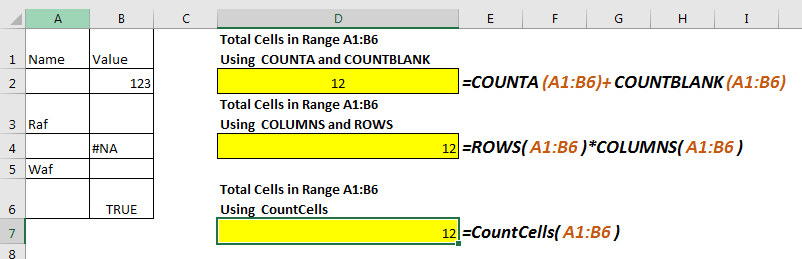There is no individual function in excel that returns total count of cells in a given range. But this doesn’t mean we can’t count all cell in excel range. Let’s explore some formulas for counting cells in a given range.
Using COUNTA and COUNTBLANK to Count Cells in a Range
*this method has problem.
As we know that t COUNTA function in excel counts any cell which is not blank. On the other hand COUNTBLANK function counts blank cell in a range. Yes, you guessed it write, we can add them to get total number of cells.
Generic Formula to Count Cells

=COUNTA(range)+COUNTBLANK(range)

Example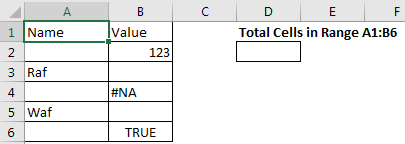Suppose, I want to to count total number of cells in range A1:B6. We can see that it has 12 cells. Now let’s use the above formula for counting the cell in given range.

=COUNTA(A1:B6)+COUNTBLANK(A1:B6)

This formula to get cell count in range returns the correct answer.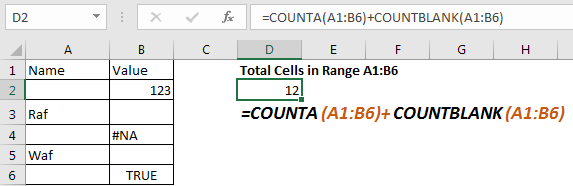The problem: If you read about COUNTA function, you’ll find that it counts any cell containing anything, even a formula that returns blank. COUNTBLANK function also counts any cell which is blank, even if it is returned by a formula. Now since both functions will count same cells, the returned value will be incorrect. So, use this formula to count cells, when you are sure that no formula returns blank value.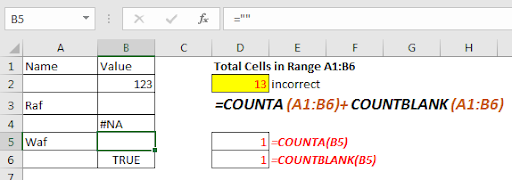Count cells in a range using ROWS and COLUMN Function
Now, we all know that a range is made of rows and columns. Any range has at least one column and one row. So, if we multiply rows with columns, we will get our number of cell in excel range. This is same as we used to calculate the area of a rectangle.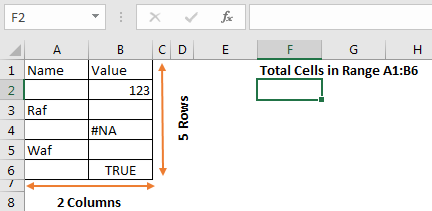Generic Formula to Count Cells

=ROWS(range)*COLUMNS(range)

Let’s implement this formula in above range to count cells.

=ROWS(A1:B6)*COLUMNS(A1:B6)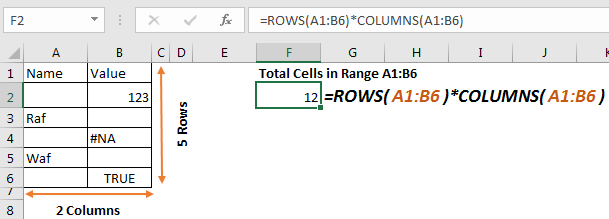This returns the accurate number of cells in range a1:B6. It doesn’t matter what values these cells hold.
How it works
It is simple. The ROWS function, returns count of rows in range, which is 6 in this case. Similarly, COLUMN function returns the number of columns in the range, which is 2 in this example. The formula multiplies and returns the final result as 12.
CountCells VBA Function to Count All Cells in a Range
In both of the above methods, we had to use two function of excel, and provide the same range twice. This can lead to be human error. So we can define a user defined function to count cells in a range. This is easy.
Press ALT+F11 to open VBA editor. Go to insert and click on module. Now copy below VBA code in that module.

```Function CountCells(rng As Range)
CountCells = rng.CountLarge
End Function```

Return to your excel file. Write CountCells function to count cells in a range. Provide range in parameter. The function will return the number of cell in the given range.

Let’s take the same example.

Write below formula to count cells in range A1:B6

=CountCells(A1:B6)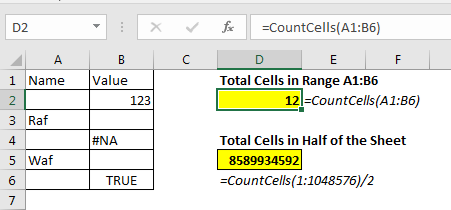How it works

Actually, CountLarge is method of range object that counts the cell in a given range. CountCells function takes the range from user as argument and returns the cell count in range using Range.CountLarge.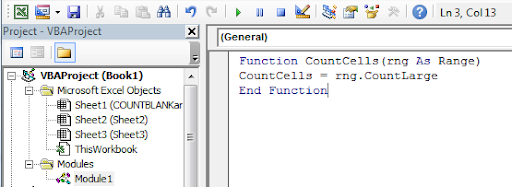This method is faster and easy to use. Once you define this function for counting cells in a range in excel, you can use it as many times as you want.

So yeah, these are the formulas to count cells in a range in excel. If you know any other ways, let us know in the comments section below.

Related Articles:

How to Count Cells that contain specific text in Excel

How to Count Unique Values In Excel

How to use the COUNT Function in Excel

How to Count Cells With Text in Excel

How to use the COUNTIFS Function in Excel

Get the Count of table rows & columns in Excel

Popular Articles :

50 Excel Shortcut to Increase Your Productivity : Get faster at your task. These 50 shortcuts will make you work even faster on Excel.

How to use the VLOOKUP Function in Excel : This is one of the most used and popular functions of excel that is used to lookup value from different ranges and sheets.

How to use the COUNTIF function in Excel : Count values with conditions using this amazing function. You don't need to filter your data to count specific values. Countif function is essential to prepare your dashboard.

How to use the SUMIF Function in Excel : This is another dashboard essential function. This helps you sum up values on specific conditions.

Terms and Conditions of use

The applications/code on this site are distributed as is and without warranties or liability. In no event shall the owner of the copyrights, or the authors of the applications/code be liable for any loss of profit, any problems or any damage resulting from the use or evaluation of the applications/code.